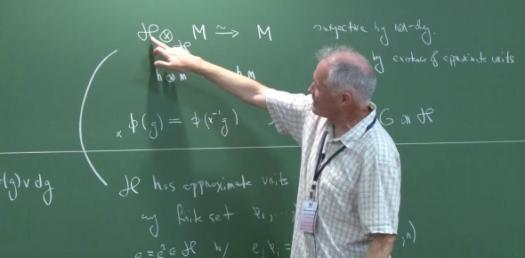# What Do You Know About Hecke Algebra?

10 Questions | Total Attempts: 102SettingsHecke algebra refers to several algebras such as Iwahori–Hecke algebra of a Coxeter group, Hecke algebra of a pair, Hecke algebra of a finite group, Hecke algebra acting on modular forms, Hecke algebra of a locally compact group. See if you can ace this quiz on the almighty Hecke algebra.

• 1.
Which Hecke algebra can be used to prove Macdonald's term conjecture for Macdonald polynomials?
• A.

Affine

• B.

Weyl

• C.

Parabolic

• D.

Paraholic

• 2.
What is an abstract group that admits a formal description in terms of reflections  or kaleidoscopic mirrors?
• A.

Symmetry group

• B.

Affine group

• C.

Coxeter group

• D.

Weyl group

• 3.
A polyhedron whose symmetry group acts transitively on its flags is?
• A.

Conic

• B.

Concave

• C.

Irregular

• D.

Regular

• 4.
What is a geometric setting in which two parameters are required to determine the position of a point?
• A.

Plane

• B.

Two-dimensional space

• C.

Bi-plane

• D.

Slope

• 5.
What is a well-behaved function from a topological group to the complex numbers which is invariant under the action of a discrete subgroup of the topological group?
• A.

Finitive group

• B.

Compact group

• C.

Automorphic form

• D.

Cosets

• 6.
A net in a ring that acts as a substitute for an identity element is?
• A.

A net identity

• B.

An approximate identity

• C.

A limit identity

• D.

An approximate limit

• 7.
If a collection of elements of a star-algebra is closed under the involution operation, it is?
• A.

Linear

• B.

Involuted

• C.

• D.

• 8.
A linear operator on a Hilbert space is called self-adjoint if it is equal to its own?
• A.

• B.

Inverse

• C.

Involution

• D.

Operator

• 9.
What is a group that is also a differentiable manifold, with the property that the group operations are compatible with the smooth structure?
• A.

Compact group

• B.

Lie group

• C.

Hecke group

• D.

Compact projector

• 10.
An isomorphism of projective spaces, induced by an isomorphism of the vector spaces from which the projective spaces derive is a?
• A.

Synthetic field

• B.

Pair group

• C.

Homography

• D.

Lie group

Related TopicsBack to top